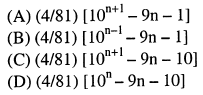Click to Answer GATE Question of the Day

### GATE Question of the Day - General Aptitude Question

The sum of n terms of the series 4+44+444+------ isVamshi said...

option C....just take 4 as common and take the g.p of the terms ... we will get the answer..Anonymous said...

It's C
Its just as easy as substituting value of 'n' and checking options.

Vamshi said...

if it is right post me...

Vamshi said...

yaaa checking is easy... bt we can do it through process also

SouravMani said...

ya from my knowledge answer is "c"! but i have doubt on this?

Vamshi said...

dude may i know what is that?

Unknown said...

yah it is C because it is in the geometric progression

SouravMani said...

hey after a stong confirmation i found the right answer the same option "C"

Unknown said...

option c......
simple trick is that put only n=o,then we see that all term become zero.which only in case option c.so don't waste your time use short trick.Anonymous said...

Option C

Unknown said...

yaa... put n=o.. den only in c ol terms wil be zero... so ans. is "C"Anonymous said...

ans iz c

Examsavvy said...

Answer to this question is option Clucky said...

this question is very easy.put n=1 then u will get the 4...so option cAnonymous said...

sum= 4+44+444+.........+tn
sum= 4+ 44+.........+tn-1+tn

subtracting above two equations we get

0=4+40+400+.............+(tn-tn-1)-tn
tn=(4+40+400+...n terms)
tn=4*(10^(n)-1)/(10-1) -- G.P summation
sum=sigma of tn
sum=4/9*(sigma of 10^(n)-sigma of 1)
sum=4/9*[10*(10^(n)-1)/(10-1)-n]
sum=option 'C'